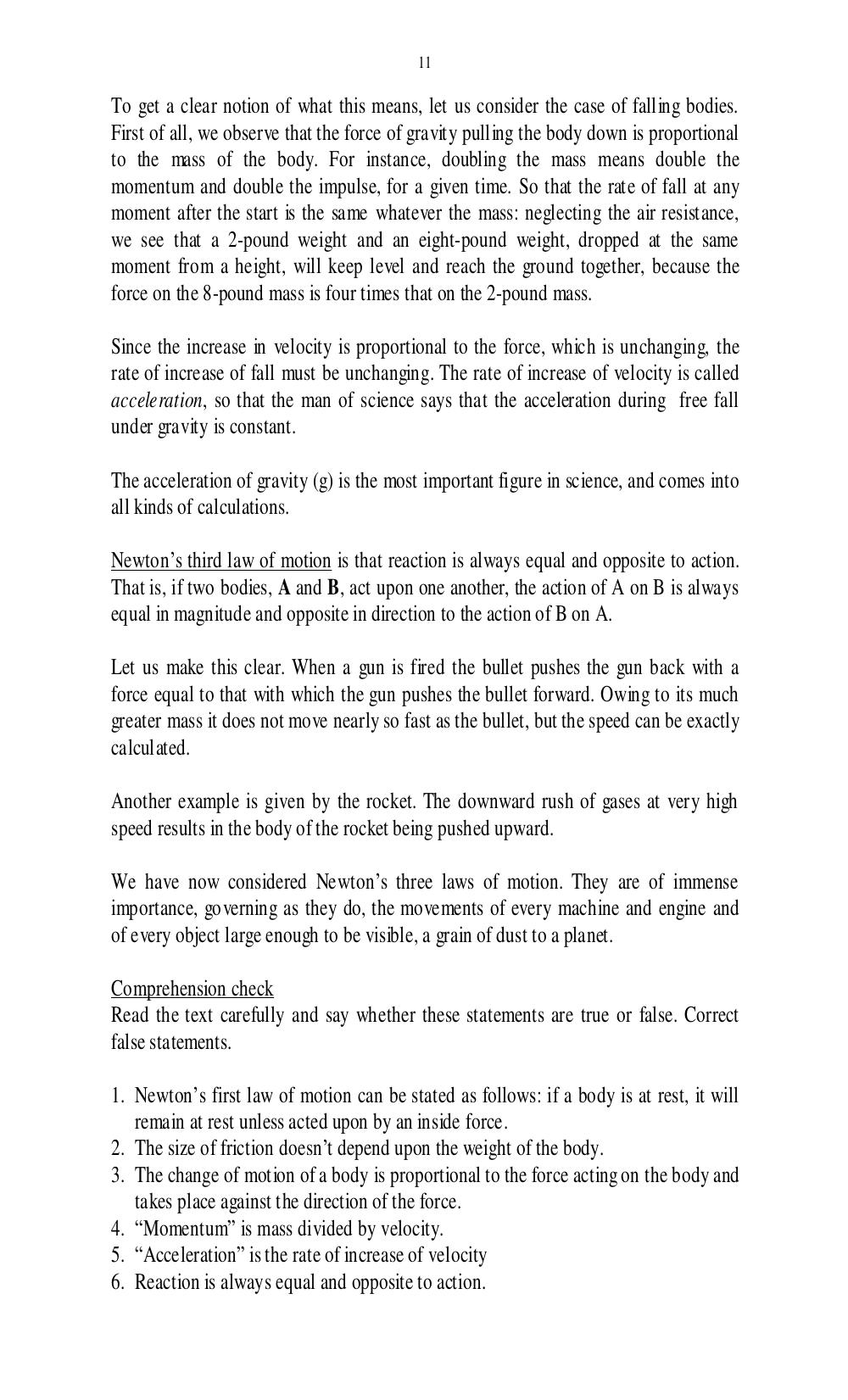# Учебно-методическое пособие по чтению специальной литературы для студентов 1 курса физического факультета. Дроздова И.В - 11 стр.

Составители:

Рубрика:

• ## Иностранный язык11
To get a clear notion of what this means, let us consider the case of falling bodies.
First of all, we observe that the force of gravity pulling the body down is proportional
to the mass of the body. For instance, doubling the mass means double the
momentum and double the impulse, for a given time. So that the rate of fall at any
moment after the start is the same whatever the mass: neglecting the air resistance,
we see that a 2-pound weight and an eight-pound weight, dropped at the same
moment from a height, will keep level and reach the ground together, because the
force on the 8-pound mass is four times that on the 2-pound mass.
Since the increase in velocity is proportional to the force, which is unchanging, the
rate of increase of fall must be unchanging. The rate of increase of velocity is called
acceleration, so that the man of science says that the acceleration during free fall
under gravity is constant.
The acceleration of gravity (g) is the most important figure in science, and comes into
all kinds of calculations.
Newtons third law of motion is that reaction is always equal and opposite to action.
That is, if two bodies, A and B, act upon one another, the action of A on B is always
equal in magnitude and opposite in direction to the action of B on A.
Let us make this clear. When a gun is fired the bullet pushes the gun back with a
force equal to that with which the gun pushes the bullet forward. Owing to its much
greater mass it does not move nearly so fast as the bullet, but the speed can be exactly
calculated.
Another example is given by the rocket. The downward rush of gases at very high
speed results in the body of the rocket being pushed upward.
We have now considered Newtons three laws of motion. They are of immense
importance, governing as they do, the movements of every machine and engine and
of every object large enough to be visible, a grain of dust to a planet.
Comprehension check
Read the text carefully and say whether these statements are true or false. Correct
false statements.
1. Newtons first law of motion can be stated as follows: if a body is at rest, it will
remain at rest unless acted upon by an inside force.
2. The size of friction doesnt depend upon the weight of the body.
3. The change of motion of a body is proportional to the force acting on the body and
takes place against the direction of the force.
4. Momentum is mass divided by velocity.
5. Acceleration is the rate of increase of velocity
6. Reaction is always equal and opposite to action.11

To get a clear notion of what this means, let us consider the case of falling bodies.
First of all, we observe that the force of gravity pulling the body down is proportional
to the mass of the body. For instance, doubling the mass means double the
momentum and double the impulse, for a given time. So that the rate of fall at any
moment after the start is the same whatever the mass: neglecting the air resistance,
we see that a 2-pound weight and an eight-pound weight, dropped at the same
moment from a height, will keep level and reach the ground together, because the
force on the 8-pound mass is four times that on the 2-pound mass.

Since the increase in velocity is proportional to the force, which is unchanging, the
rate of increase of fall must be unchanging. The rate of increase of velocity is called
acceleration, so that the man of science says that the acceleration during free fall
under gravity is constant.

The acceleration of gravity (g) is the most important figure in science, and comes into
all kinds of calculations.

Newton’s third law of motion is that reaction is always equal and opposite to action.
That is, if two bodies, A and B, act upon one another, the action of A on B is always
equal in magnitude and opposite in direction to the action of B on A.

Let us make this clear. When a gun is fired the bullet pushes the gun back with a
force equal to that with which the gun pushes the bullet forward. Owing to its much
greater mass it does not move nearly so fast as the bullet, but the speed can be exactly
calculated.

Another example is given by the rocket. The downward rush of gases at very high
speed results in the body of the rocket being pushed upward.

We have now considered Newton’s three laws of motion. They are of immense
importance, governing as they do, the movements of every machine and engine and
of every object large enough to be visible, a grain of dust to a planet.

Comprehension check
Read the text carefully and say whether these statements are true or false. Correct
false statements.

1. Newton’s first law of motion can be stated as follows: if a body is at rest, it will
remain at rest unless acted upon by an inside force.
2. The size of friction doesn’t depend upon the weight of the body.
3. The change of motion of a body is proportional to the force acting on the body and
takes place against the direction of the force.
4. “Momentum” is mass divided by velocity.
5. “Acceleration” is the rate of increase of velocity
6. Reaction is always equal and opposite to action.